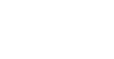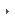UOM

 Quicklinks eSIMS Campus FM Coordinating Events Degree Plus Webmail Library OPAC Job Opportunities E-Learning Activate IT Services Student Account

 CHE1200: Mathematics for ChemistsFaculty HomepageDepartment HomepageProf. JN Grima - HomeTeachingResearchPublicationsOther Academic ActivitiesPersonal PagesLinks01 | 02 | 03 | 04 | 05 | 06 | RESOURCES

CHE1200 - Syllabus:

NOTE: This local version is NOT the offical version of the CHE1200 syllabus.
The official version is the one on e-sims.

 Credit Value: 4 ECTS No. of Lectures: 28 No. of Tutorials/Labs: 28 Year: 1 Semesters: 1 & 2 Pre-requisite: O-Level Maths

1. Elementary algebra:
• Evaluation of expressions: Introduction to the f(x) notation, brackets, factorising, solving quadratic equations, solving simultaneous equations, partial fractions, inequalities, sigma and pi notation
• Functions: trigonometric, exponential, logarithmic, inverse functions,
• Essential co-ordinate geometry
• Complex numbers
• Series

2. Calculus (Theory):
• Differentiation: Differentiation of basic functions, product rule, quotient rule, minima and maxima, function of a function, chain rule, curve sketching and essential coordinate geometry, complex numbers, Maclaurin and Taylor Series.
• Integration: Integration of basic functions. Integration by substitution, integration by parts, finite integration, numerical integration.
• Functions of several variables and partial differentiation.
• Differential equations: First and second order differential equation, boundary conditions

3. Calculus (Applications):
• Application of differentiation to locate and identify turning points.
• The role of calculus in thermodynamics.
1. The use of integration to calculate p-V work (i.e. to find the area under p-V graphs).
2. Integration as a means to obtain a 'measurable' change in a quantity, delta_x, from infinitesimally small changes, dx.
3. Partial differentiation and state functions.
4. The use of partial derivatives to differentiate expressions of the sort G = H- TS, and then use these to find how, for example, G varies with T at constant p.
5. The role of differential equations in:
• Chemical kinetics;
• Quantum mechanics.

4. Matrices and determinants:
• Matrix notation
• Elementary matrix operations and properties
• Determinants
• Inverse matrices
• Eigenvalues and eigenvectors

5. Probability and statistics:
• Permutations and combinations
• Introduction to statistics
• Regression analysis
• Applications

6. Mathematics through computers:
• Plotting of curves, data analysis, etc.

Method of assessment:

Course work: 25%
End of year exam: 75%

Last Updated: 03rd October, 2007